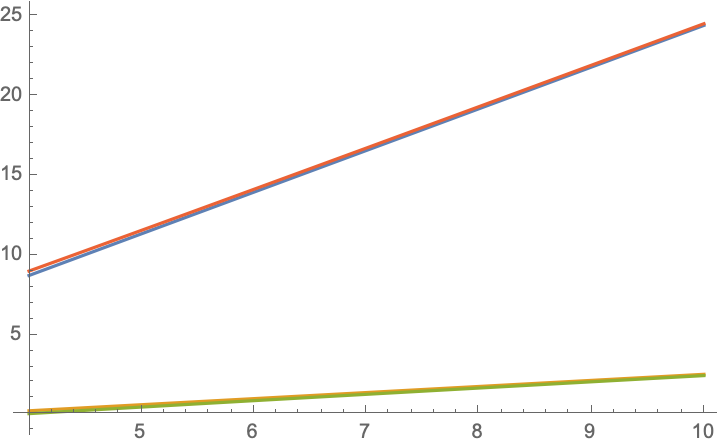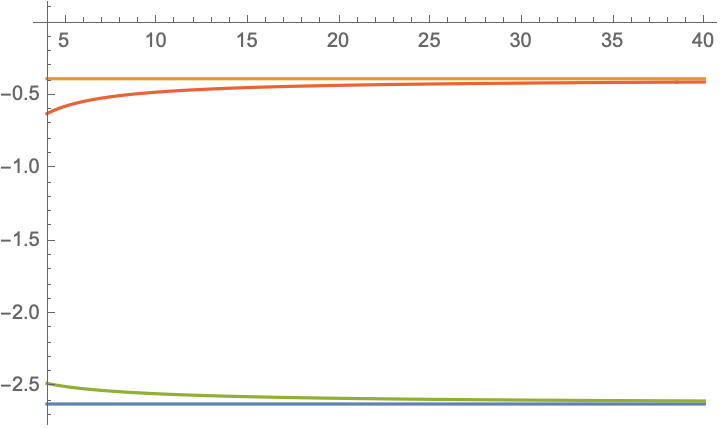# How to find bounds on the eigenvalues of a matrix?

Given this matrix

$$M=\begin{bmatrix} 0 & m-1 & 2 & n-1\\ 1 & m-2& 1 & n-1\\ 2 & m-1 & 0 & 2(n-1)\\ 1 & m-1 & 2 & 2(n-2)\end{bmatrix},$$ show that if $$\alpha_1,\alpha_2$$ are two negative eigenvalues of $$M$$, then $$\alpha_1+\alpha_2<-3$$. Alsoo $$m,n\ge 4$$ are integers.

Using Gershgorin circles theorem, the eigenvalues would lie in the intervals $$B(0,m+n)$$, $$B(m-2,n-1)$$, $$B(0,2n+m-1)$$,$$B(2n-4,m+2)$$ where $$B(x,y)$$ denotes the interval with centre $$x$$ and radius $$y$$. But it's not helping to find the required bound?

Does there exist any theorem/trick to find a better upper bound of the sum of two negative eigenvalues of a matrix?

If someone can help, I will be grateful.

• What are the constraints on $m$ and $n$? Are they integers? non-negative (or positive) integers?
– gmvh
Feb 8, 2021 at 16:26
• @gmvh; I have edited it Feb 8, 2021 at 16:51
• If this is still relevant, you can use Tarski-Seidenberg to get a complete answer for real $m,n$ in any specified range. Together with an explicit computation for small integer values of $m,n$, this should probably suffice. Apr 16, 2021 at 14:20

I am able to accurately calculate the eigenvalues in perturbation theory, as follows:

I perform a similarity transformation on the matrix $$M$$, given by $$M'=S^{-1}MS$$ with $$S=\left( \begin{array}{cccc} 1 & 1 & \frac{-\sqrt{m^2+4 n^2}+m-2 n}{2 m} & \frac{\sqrt{m^2+4 n^2}+m-2 n}{2 m} \\ 0 & 0 & \frac{-\sqrt{m^2+4 n^2}+m-2 n}{2 m} & \frac{\sqrt{m^2+4 n^2}+m-2 n}{2 m} \\ \frac{1}{2} \left(-\sqrt{5}-1\right) & \frac{1}{2} \left(\sqrt{5}-1\right) & 1 & 1 \\ 0 & 0 & 1 & 1 \\ \end{array} \right).$$ The matrix $$M'$$ has the same eigenvalues as $$M$$ and is given by $$M'=M_0+M_1$$, with $$M_0=\left( \begin{array}{cccc} 0 & 0 & 0 & 0 \\ 0 & 0 & 0 & 0 \\ 0 & 0 & \frac{1}{2} \left(-\sqrt{m^2+4 n^2}+m+2 n\right) & 0 \\ 0 & 0 & 0 & \frac{1}{2} \left(\sqrt{m^2+4 n^2}+m+2 n\right) \\ \end{array} \right),$$ $$M_1=\left( \begin{array}{cccc} \frac{1}{2} \left(-\sqrt{5}-3\right) & 0 & \frac{\sqrt{m^2+4 n^2}+\left(\sqrt{5}-2\right) m+2 n}{2 \sqrt{5} m} & \frac{-\sqrt{m^2+4 n^2}+\left(\sqrt{5}-2\right) m+2 n}{2 \sqrt{5} m} \\ 0 & \frac{1}{2} \left(\sqrt{5}-3\right) & \frac{-\sqrt{m^2+4 n^2}+\left(\sqrt{5}+2\right) m-2 n}{2 \sqrt{5} m} & \frac{\sqrt{m^2+4 n^2}+\left(\sqrt{5}+2\right) m-2 n}{2 \sqrt{5} m} \\ \frac{-\sqrt{5} \left(\sqrt{m^2+4 n^2}-2 n\right)-m}{2 \sqrt{m^2+4 n^2}} & \frac{\sqrt{5} \left(\sqrt{m^2+4 n^2}-2 n\right)-m}{2 \sqrt{m^2+4 n^2}} & -\frac{3 \sqrt{m^2+4 n^2}+m-2 n}{2 \sqrt{m^2+4 n^2}} & -\frac{\sqrt{m^2+4 n^2}+m-2 n}{2 \sqrt{m^2+4 n^2}} \\ \frac{m-\sqrt{5} \left(\sqrt{m^2+4 n^2}+2 n\right)}{2 \sqrt{m^2+4 n^2}} & \frac{\sqrt{5} \left(\sqrt{m^2+4 n^2}+2 n\right)+m}{2 \sqrt{m^2+4 n^2}} & \frac{-\sqrt{m^2+4 n^2}+m-2 n}{2 \sqrt{m^2+4 n^2}} & \frac{-3 \sqrt{m^2+4 n^2}+m-2 n}{2 \sqrt{m^2+4 n^2}} \\ \end{array} \right).$$

Now we note that for large $$m,n$$ of order $$1/\epsilon$$, the matrix $$M_0$$ is of order $$1/\epsilon$$ while the matrix $$M_1$$ is of order unity. We can therefore perform perturbation theory in the small parameter $$\epsilon$$.

Let me first look at the positive eigenvalues. To zeroth order these are given by $$\beta_\pm=\frac{1}{2} \left(\pm \sqrt{m^2+4 n^2}+m+2 n\right)$$. To first order these are the $$(3,3)$$ and $$(4,4)$$ diagonal elements of $$M_0+M_1$$, which gives the two positive eigenvalues $$\beta_\pm=\frac{1}{2} \left(m+2 n\pm\sqrt{m^2+4 n^2}\right)\pm\frac{m-2 n \mp 3 \sqrt{m^2+4 n^2}}{2 \sqrt{m^2+4 n^2}}.$$ This expression for the positive eigenvalues is quite accurate, see the plot for $$m=n$$, where the exact expressions (red and green lines) are almost indistinguishable from the perturbative result (blue and orange).Now for the negative eigenvalues. To first order these are given by the $$(1,1)$$ and $$(2,2)$$ diagonal elements of $$M_0+M_1$$, equal to $$\alpha_1=\frac{1}{2} \left(-\sqrt{5}-3\right)=-2.618,\;\;\alpha_2=\frac{1}{2} \left(\sqrt{5}-3\right)=-0.382.$$

Here is the plot for $$m=n$$, again red and green is exact, blue and orange the perturbative result.So to first order in $$\epsilon$$ one has $$\alpha_1+\alpha_2=-3$$. The challenge is to prove that higher order corrections are negative. Incidentally, the restriction to integer $$m,n$$ does not seem to play any role (in the plot $$m=n$$ is varied continuously).

• I am thankful for such a detailed explanation, I would be grateful if you could explain how you obtained the two matrices $M_0,M_1$ Feb 9, 2021 at 13:48
• this is the usual way one does perturbation theory: you first look only at the elements of $M$ that grow with increasing $m,n$, that matrix can be diagonalized by $S$ and that gives $M_0$; then what is left is a correction $M_1$, so $S^{-1}MS=M_0+M_1$. Feb 9, 2021 at 13:56
• I was just wondering if this can be done with any bounds that exist on smallest and second smallest eigenvalue of a matrix, it just came to my mind so I thought of sharing with you Feb 9, 2021 at 14:00
• just for my information: why do you insist on this inequality, isn't the knowledge of the eigenvalues themselves of interest? as you can see the perturbative results are quite accurate. Feb 9, 2021 at 14:37
• @user64494 --- $\alpha_1$ and $\alpha_2$ are independent of $n,m$, equal to $-\tfrac{1}{2}(3\pm\sqrt 5)$, when both $n$ and $m$ are $\gg 1$. Feb 9, 2021 at 23:01

This can be done with the help of Mathematica. First, the function $$f(m,n)$$ finds the sum of the negative eigenvalues of the matrix under consideration. Second, we numerically find the maximum value of $$f(x,y)$$ over the integers greater than or equal to $$4$$ with relative error $$10^{-3}$$. The results are as follows.

f[m_, n_] := Total[Map[Min[0, #] &,
Eigenvalues[{{0, m - 1, 2, n - 1}, {1, m - 2, 1, n - 1}, {2, m - 1,
0, 2 n - 2}, {1, m - 1, 2, 2 n - 4}}] // N]]
NMaximize[{f[x, y],   x >= 4 && y >= 4 && x \[Element] Integers && y \[Element] Integers},
{x, y}, Method -> {"DifferentialEvolution", "ScalingFactor" -> 1},
AccuracyGoal -> 3, PrecisionGoal -> 3, MaxIterations -> 1000]


$$\{-3.,\{x\to 12854143321479777526031848555457428151289960383333269504,y\to 1051298338250582241682620486779844006807195121763745792\}\}$$ Edit. A typo in the code for the matrix under consideration.

• For example, n = 61; m = 56; Eigenvalues[{{0, m - 1, 2, n - 1}, {1, m - 2, 1, n - 1}, {2, m - 1, 0, 2 n - 2}, {1, m - 1, 2, 2 n - 2}}] // N performs $\{155.857,21.113,-2.58165,-0.388454\}$. Feb 8, 2021 at 20:53
• but $-2.67282$ is greater than $-3$, so you are saying the claim of the OP is false? the value for $m$ where this sum is reached seems so astronomically large, it looks suspiciously like an issue of too little numerical accuracy Feb 8, 2021 at 21:08
• @CarloBeenakker: Yes, f[62605783003825957106601552641839576616084246664423713800192, 4] produces $-2.67282$ and m = 62605783003825957106601552641839576616084246664423713800192; n = 4;Eigenvalues[{{0, m - 1, 2, n - 1}, {1, m - 2, 1, n - 1}, {2, m - 1, 0, 2 n - 2}, {1, m - 1, 2, 2 n - 2}}] // N results in $\{155.857,21.113,-2.58165,-0.388454\}$. Feb 8, 2021 at 21:27
• the matrix of the OP has $2(n-2)$ and not $2n-2$ as in your Mathematica code Feb 8, 2021 at 21:31
• @user64494; thanks for your efforts, but the problem needs to be proved mathematically and not through any software like Mathematica Feb 9, 2021 at 2:28